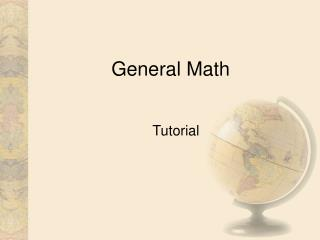DownloadDownload PresentationGeneral Math

# General Math

Télécharger la présentation## General Math

- - - - - - - - - - - - - - - - - - - - - - - - - - - E N D - - - - - - - - - - - - - - - - - - - - - - - - - - -
##### Presentation Transcript

1. General Math Tutorial

2. General Math • General Math consists of things you do in your everyday life. In this tutorial, we will cover Weights and Measures, Area and Volume, and Conversions. In most situations, you will not be required to memorize the formulas, as they will be given to you. After all, in the real world, you would be able to look them up whenever you needed. Most people don’t convert Fahrenheit to Celsius everyday, but you should know how.

3. General Math • Weights and Measures • Conversions between U.S. and Metric Measurements confront us almost daily. So let’s try a few. • 1 foot = 12 inches • 1 yard = 3 feet • Now what if we want to know how many inches are in 8 yards? • Just like the word problems, we break it down into what we know and what we don’t know.

4. General Math • We know we have 8 yards. And we know there are 3 feet in 1 yard. So… • 8 x 3 = 24 • There are 24 feet in 8 yards. • Now we know that 1 foot = 12 inches. So… • 24 x 12 = 288 • There are 288 inches in 24 feet. • Thus…. 8 yards = 24 feet = 288 inches or 8 yards = 288 inches

5. General Math • Now let’s try another problem using weight. Given that: • 1 pound (lb) = 16 ounces (oz) • 1 pound (lb) = 0.454 kilograms (kg) - (**This is an approx. weight but will be used for this entire lesson.)

6. General Math • How many kilograms are there in 112 ounces? • If there are 16 oz. In 1 lb., then how many pounds does 112 oz. Make? Or, how many times does 16 go into 112? • 112 / 16 = 7 lbs • Now if 1 lb is approximately equal to 0.454 kg, how many kg do we have with 7 lbs? • 0.454 x 7 = 3.178 kg. • So…. 112 oz = 7 lbs = 3.178 kg or 112 oz = 3.178 kg

7. General Math • Conversions • Now that you’ve had some experience with conversions using weights and measures, let’s apply that to other things. • Here is the formula for converting Fahrenheit to Celsius and vice versa. • To convert Fahrenheit (°F) to Celsius (°C), subtract 32 and divide by 1.8 • To convert Celsius (°C) to Fahrenheit (°F), multiply by 1.8 and add 32

8. General Math • Now that you have the formula, what would 5 °C be in Fahrenheit? • We are converting °C to °F, so… • 1.8 x 5 = 9.0 • 9.0 + 32 = 41 °F • Brrr…It’s gonna be chilly. Might want a jacket today! • Let’s try another one. Convert 34 °C to °F • 1.8 x 34 = 61.2 • 61.2 + 32 = 93.2 °F

9. General Math • Now it’s time to find the air conditioner! • One more…but let’s try the other way now. Convert 90 °F to °C • 90 – 32 = 58 • 58 / 1.8 = 32.2 °C • Nice job!

10. General Math • Area and Volume • Time for some area calculations now. First we must know that when calculating the area of a square or rectangle, the standard formula is • Area = Length x Width • Also, to represent area, we always use square units to express our answer. • So a rectangle that is 10 inches wide and 15 inches long would be calculated like this

11. General Math • Area = 15 x 10 • Area = 150 square inches • What about a room that is 10 feet wide and 10 feet long? • Area = 10 x 10 ….or 100 square feet

12. General Math • Don’t sweat it. You’ll always be given the formula in the tests for our lessons. Again, we don’t expect you to memorize all this…just to know how to do it. • Look’s like you’re ready to conquer the test for this module! • Good Luck!

13. General Math You must make a perfect score of 100% to pass. Click here to take the test for this module.